# Pump Related formulas | Power calculation, Total Head, NPSH, Affinity laws

In this article provided pump related formulas like fluid flow rate and velocity, power calculation, Specific Speed of Pump (Nq), Total Head, Pump Torque and temperature rise, Net Positive Suction Head, Affinity laws for pump, Pump Efficiency & Overall Efficiency of the Pump

## Basic required formulas for the operation of Pump

### Formulas regarding product while pumping

Volume of the fluid (Q )Velocity of the Fluid ( V )Here

V = Velocity of fluid in m/sec
Q =Volume of Fluid (m3/sec)
A = Pipe line area (m2)V = Velocity of fluid in m/sec
Q =Volume of Fluid in m3/hr
A = Pipe line dia in mm

Reynolds Number of the fluidHere
D = Dia of the tube in meters

V = flui
d velocity in m/sec
ρ=
density of the fluid  (kg/m3)
μ = Absolute viscosity in Pas

Absolute viscosity of the fluid (μ )

μ υ x ρ

where:
υ = Kinematic viscosity (mm
2/s)
ρ = density of the fluid in kg/m3

μ = Absolute viscosity of the fluid in mPas

Darcy Friction Factorwhere:
fd
= friction factor (Darcy)
Re = Reynolds number

### Pump Power Calculation

Hydraulic Pump Power

The ideal hydraulic power to drive a pump depends on liquid density , differential height to lift the material and flow rate of the material.Here

Hydraulic power in watt

Q = Flow rate in M3/sec

ρ = Density of the Fluid kg/dm3 (1 kg/m3 = 0.001 kg/dm3)

g = Acceleration due to gravity (m/sec2)

Pump Power input or Pump shaft Power

The pump power input of a centrifugal pump is the mechanical energy at the pump coupling or pump shaft absorbed from the drive.Here

Q = Flow rate in M3/sec

ρ = Density of the Fluid ( kg/dm3)

ηp =Pump efficiencyHere

Q = Flow rate in M3/hr

ρ = Density of the Fluid ( kg/dm3 )

ηp =Pump efficiency

Pump input power from pump shaft powerPump input power from current and voltageHere all efficiencies are in decimals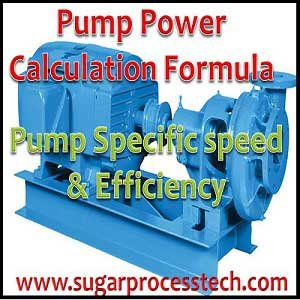### Specific Speed of Pump (Nq)

Specific Speed of pump (Nq) is identifies the geometrical similarity of pumps. It is useful to comparing different pump designs irrespective of pump size

Nq ==Where Nq = Dimensionless parameter

N = RPM of pump

n = Rev/sec of Pump

Q =  Flow rate in m3/sec

g = Gravitational constant ( 9.81 m/sec2)

Suction Specific Speed at best efficiency pointNs = Suction Specific speed of pump (Dimensionless parameter)

N = RPM of the pump

Q = Flow rate in M3/hr

NPSH = Net positive section head in meters

In pumping system, Head means it is a height of a liquid coloumn.

In vertical pipe any liquid coloumn of water exerts a certain pressure (force per unit area) on a horizontal surface at the bottom area, this pressure is expressed in metres of liquid column or kg/cm2.

H = Ht (±Hs)

where:
H
H

Ht = ht + hft + pt

where:
h
h
ft = pressure drop in discharge line

pt = Pressure head in delivery
p
t > 0 for pressure
p
t < 0 for vacuum
p
t = 0 for open tank

Hs = hs + hfs + (± ps)

where:
h
hfs = pressure drop in suction line

ps = Pressure head in suction
p
s > 0 for pressure
p
s < 0 for vacuum
p
s = 0 for open tank

Pressure Head of the pump suction must be considered according to the condition of source tank.

Pressure head calculated as per pumping system source tank is under some gauge pressure or vacuum open or open to atmospheric than pressure head is calculated in metres of water column (MWC) of Feet of water column of liquid.Here Hv = Velocity head in meters

V = Fluid velocity in m/sec

g = Gravitational constant (9.81 m/s2)

Q = Flow rate in m3/hr

d = pipe inside diameter in mm

Shutoff head of the centrifugal pump is the maximum head that can be developed by a pump operating at a set speed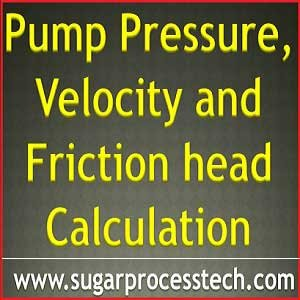### Pump Torque and temperature riseTemperature rise in pumps can be calculated as per the below formulaHere

ΔT = Temperature rise in the pump (in oC)

P = brake power (kW)

ηp =Pump efficiency

Cp = specific heat of the fluid (kJ/kg oC)

Q = Flow rate of the pump (m3/second)

ρ = fluid density (kg/m3)

Net Positive Suction Head Required (NPSHr ):

The amount of NPSH the pump requires to avoid cavitation is called Net Positive Suction Head Required (NPSHr). This value of the pump is determined based on actual pump test by the vendor.

Net Positive Suction Head Available( NPSHa) :

Net positive suction head available is the difference between the saturation pressure and the pump suction pressure for the liquid being pumped.

The amount of  Net positive suction head available (NPSHa) to the pump from the suction line is termed NPSHa.

NPSHa = Absolute Pressure head + Static head (difference in elevation) – Vapor pressure head – Friction head loss in the piping, valves and fittings.

Net positive suction head available must be greater than or equal to the net positive suction head required to avoid cavitation. It can be stated mathematically as shown below.
NPSH a NPSH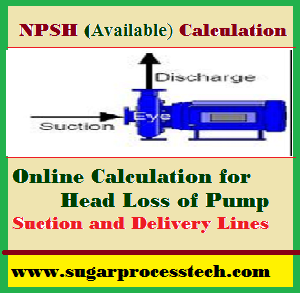Formulas of pump NPSH and head loss calculation in suction and delivery line

### Affinity laws for pumps

Change in DiameterD = Diameter of the impeller (inch or mm)

Q = Flow rate (gpm or m3/hr)

H = Head (ft or m)

P = Power ( hp or kW)

Change in SpeedN = Pump speed (RPM)

Q = Flow rate (gpm or m3/hr)

H = Head (ft or m)

P = Power ( hp or kW)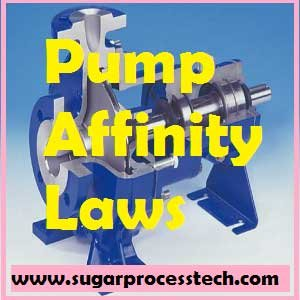### Pump Efficiency & Overall Efficiency of the Pump

Generally for any system efficiency means the ratio of output and input

Efficiency of the system =Pump Efficiency can be defined as a ratio of pump input and output power.

i.e Efficiency of the pump defined as the ratio of water horse power to break horse power.Hydraulic power in Watt =Here

Q = Flow rate in m3/sec

H = Total developed head in meters= Density in kg/m3

Hydraulic power  in kW =Here

Q = Flow rate in Lt./sec ( 1 m3/sec = 3.6 x Lt./sec)

H = Total developed head in meters= Density in kg/dm3 (Conversion : 1 kg/m3 = 0.001 kg/dm3)

Pump shaft power means it is an input to pump often is the output of motor.

In case of gear drive or pulley drive, efficiency of these drives will also have to be taken into account

Output of motor = (kW input to motor) x motor efficiency (ηm)

Input to motor is measured directly in kW.

Then
Pump efficiency ηp =Overall Efficiency of the pumping system

Overall efficiency (ηoverall) = Pump efficiency (ηp) x Motor efficiency (ηm)

Overall efficiency (ηoverall) =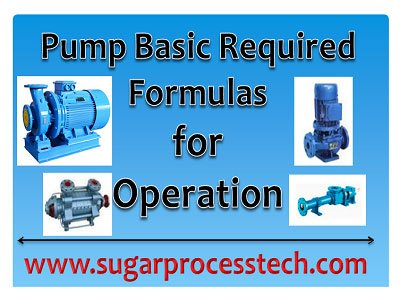Related Articles:

Classification of pumps | Types of pumps and their working principles

NPSH Calculation |Head loss in suction and delivery line

Pump Vapour pressure calculation | Water Vapour Pressure Table at Different temperatures

Affinity Laws for Centrifugal and Positive displacement pumps

Pump Efficiency and Pump Power Calculation Formulas with Online Calculator

Unit Conversion Factors and Tables for Engineering Design Calculations

## 3 thoughts on “Pump Related formulas | Power calculation, Total Head, NPSH, Affinity laws”#### joel

(September 20, 2022 - 5:11 am)

very handy to speed up calculation#### siva alluri

(October 1, 2022 - 3:31 pm)

welcome#### Debasis Maitra

(December 8, 2022 - 11:07 am)

Can we calculate head of a pump with the the help of impeller diameter or any other simple method for pump discharge head calculation.